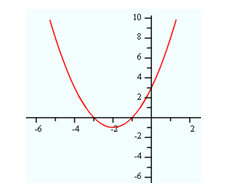Finding Zeros

In order to graph a polynomial function, knowing where the graph crosses the x-axis is necessary. The value(s) of the x-intercept(s) are the zero(s) or real root(s) of the function. To determine the zeros of a polynomial function set the equation equal to zero.

Example Find the zeros of

f(x) = x2 + 4x + 3.

Step 1. Set the equation equal to zero.

x 2 + 4x + 3 = 0

Step 2. Solve for x, in this case by factoring.

(x + 3)(x + 1) = 0

Step 2a. Set each binomial equal to zero.

x + 3 = 0 or x + 1 = 0

Step 2b. Find the values of x.

x = -3 or x = -1

The solutions found are the values where the function intersects the x-axis.*Note: the y-intercept is 3, found when x = 0 ? f(0) = 02 + 4?0 + 3 = 3.